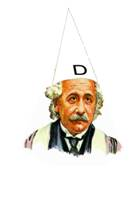An Investigation of Modern Physics by Brian Williams
• ## Einstein’s Wrong Formulae

Posted on June 26th, 2009

Do you know the important differences between these formulae?t1 = [d/(c + v)] + [d/(c – v) ] A1 t1 = [d1/(c + v )] + [d2/(c – v)] A2 t2 = 2d/(c2 – v2)0.5 B1 t2 = (d1 + d2)/(c2 + v2)0.5 B2

Formulae A1 and B1 are the ones used by physicists to ‘prove’ the constant speed of light on the assumption that they are in accordance with classical mechanics. These are the formulae given in physics books. Unfortunately, they are both “special case” formulae which are quite specifically not applicable to the Michelson – Morley experiment on which Einstein’s Relativity is based.

Formulae A2 and B2 are the ones that are correct in accordance with classical mechanics. Although the differences appear minor, Einstein’s Relativity, The Constant Speed of Light, Time Dilation, E = mc2 etc. are all based on the formulae A1 and B1.

More importantly, the above formulae are only 25% of the mathematics needed to solve the problem of the Michelson-Morley experiment. The other 75% is not even considered by Einstein and the rest of the physics establishment

Einstein, not understanding mechanics, did not realise the implications of this error of Michelson and Morley and carried the same error into his own calculations.

If the correct formulae are used i.e. A2 and B2, plus the omitted 75% , all the above hypotheses become extinct, and light is proved to strictly follow the principles of classical mechanics.

Does it matter? Yes, the use of the wrong formulae has cost the people of the UK billions of pounds, and is still costing many millions of pounds every year.

Physics or Fantasy – Section 1 details the errors in full and shows that the mathematics relating to the time of transit for the beams of light in the Michelson – Morley experiment are more complex than realised by the physicists.